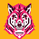# Moving Average Cross Alert, Multi-Timeframe (MTF) (by ChartArt)

41410 visitas
See when two moving averages cross. With the option to choose between four moving average calculations:

The moving averages can be plotted from different time-frames, like e.g. the weekly or 4 hour time-frame using HL2 , HLC3 or OHLC4 as price source for the calculation. In addition there is a background color alert and arrows when the moving averages cross each other when the price also rises or falls. And the moving averages are colored depending on their trend direction (if they are trending up or down).
```study("Moving Average Cross Alert, Multi-Timeframe Option (MTF) (by ChartArt)", shorttitle="CA_-_MA_cross", overlay=true)

// ChartArt's Moving Average Cross Indicator
//
// Version 1.0
// Idea by ChartArt on September 15, 2015.
//
// This indicator shows when two moving averages cross.
// With the option to choose between four moving
// average calculations:
// (SMA = simple moving average)
// (EMA = exponential moving average)
// (WMA = weighted moving average)
// (Linear = Linear regression)
//
// The moving averages can be plotted from different
// timeframes, like the weekly or 4 hour timeframe.
// With the possibility to use HL2, HLC3 or OHLC4 prices.
//
// and arrows when the moving averages cross each other.
// And the moving averages are colored depending if
// they are trending up or down.
//
// List of my work:

// Multi-timeframe and price input
pricetype = input(close, title="Price Source For The Moving Averages")
useCurrentRes = input(true, title="Use Current Timeframe As Resolution?")
resCustom = input(title="Use Different Timeframe? Then Uncheck The Box Above", type=resolution, defval="W")
res = useCurrentRes ? period : resCustom
price = security(tickerid, res, pricetype)

// MA period input
shortperiod = input(50, title="Short Period Moving Average")
longperiod = input(100, title="Long Period Moving Average")

// MA calculation
smoothinput = input(2, minval=1, maxval=4, title='Moving Average Calculation: (1 = SMA), (2 = EMA), (3 = WMA), (4 = Linear)')
short = smoothinput == 1 ? sma(price, shortperiod) :
smoothinput == 2 ? ema(price, shortperiod) :
smoothinput == 3 ? wma(price, shortperiod) :
smoothinput == 4 ? linreg(price, shortperiod,0) :
na
long = smoothinput == 1 ? sma(price, longperiod) :
smoothinput == 2 ? ema(price, longperiod) :
smoothinput == 3 ? wma(price, longperiod) :
smoothinput == 4 ? linreg(price, longperiod,0) :
na

// MA trend direction color
shortcolor = short > short ? lime : short < short ? red : blue
longcolor = long > long ? lime : long < long ? red : blue

// MA output
MA1 = plot(short, title="Short Period Moving Average", style=linebr, linewidth=2, color=shortcolor)
MA2 = plot(long, title="Long Period Moving Average", style=linebr, linewidth=4, color=longcolor)
fill(MA1, MA2, color=silver, transp=50)

// MA trend bar color
TrendingUp() => short > long
TrendingDown() => short < long
barcolor(TrendingUp() ? green : TrendingDown() ? red : blue)

MAcrossing = cross(short, long) ? short : na
plot(MAcrossing, style = cross, linewidth = 4,color=black)

// MA cross background color alert
Uptrend() => TrendingUp() and TrendingDown()
Downtrend() => TrendingDown() and TrendingUp()
bgcolor(Uptrend() ? green : Downtrend() ? red : na,transp=50)

Buy = Uptrend() and close > close
Sell = Downtrend() and close < close
plotshape(Sell, color=black, style=shape.arrowdown, text="Sell", location=location.top)
```

## ComentariosThis was just what I was looking for. Unfortunately, if I set to the 1 hour time frame and then use a 5 minute chart then it is not accurate and the same as the 1 hour ribbon. I tried both checking the box and unchecking the box and the ribbon will not display the same way on a lower time frame. With the lower time frames, the ribbon becomes choppy which defeats the purpose of using the 1 hour ribbon.
Responderhi, such a great idea and script :)

im trying to make it as a strategy for back testing

I wrote :

if (sell)
strategy.entry("sell", strategy.short)

but im getting error as ( line 43: syntax error at input 'end of line without line continuation' ) with @version3 and ( line 43: no viable alternative at input 'end of line without line continuation' ) with @subversion23